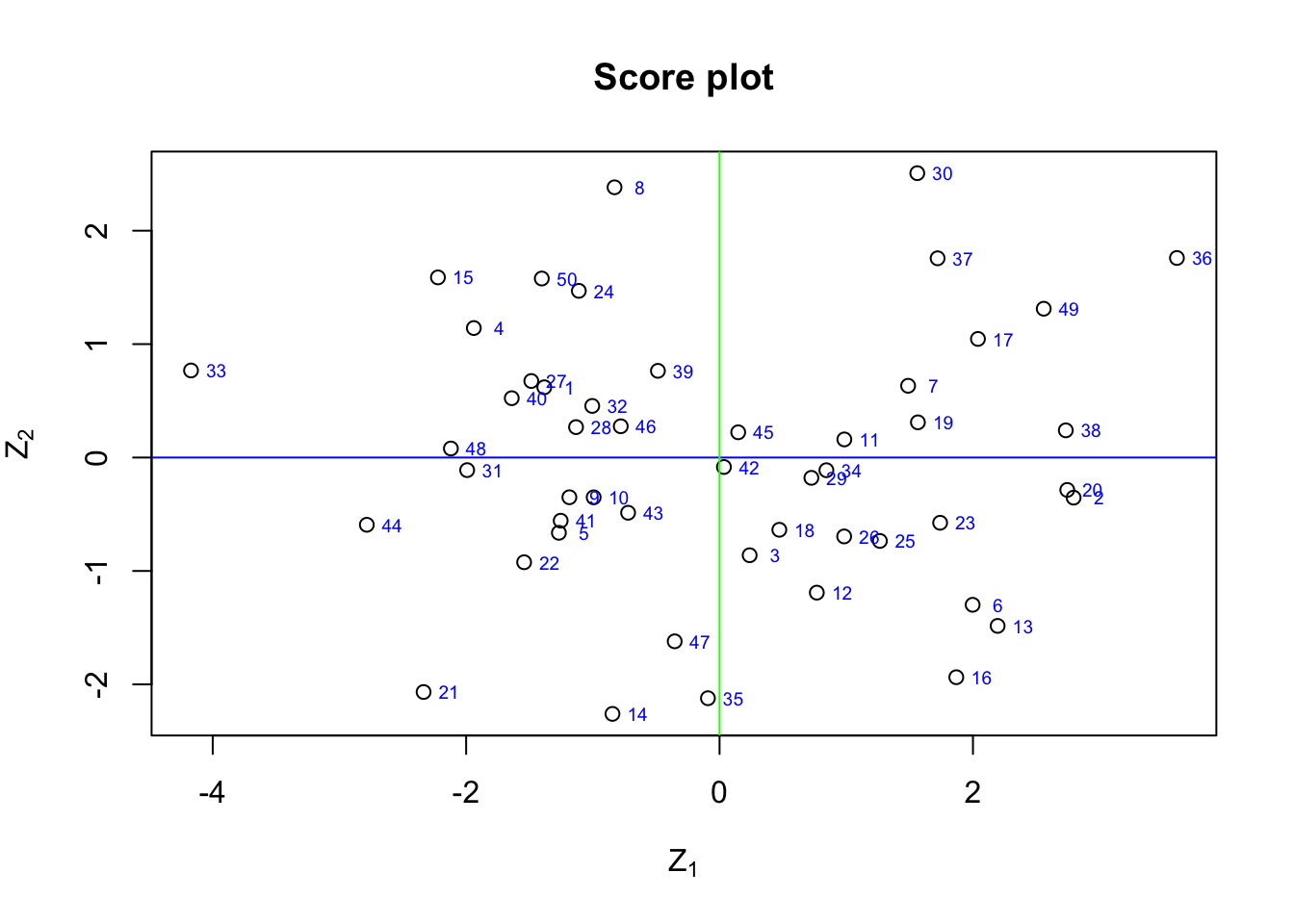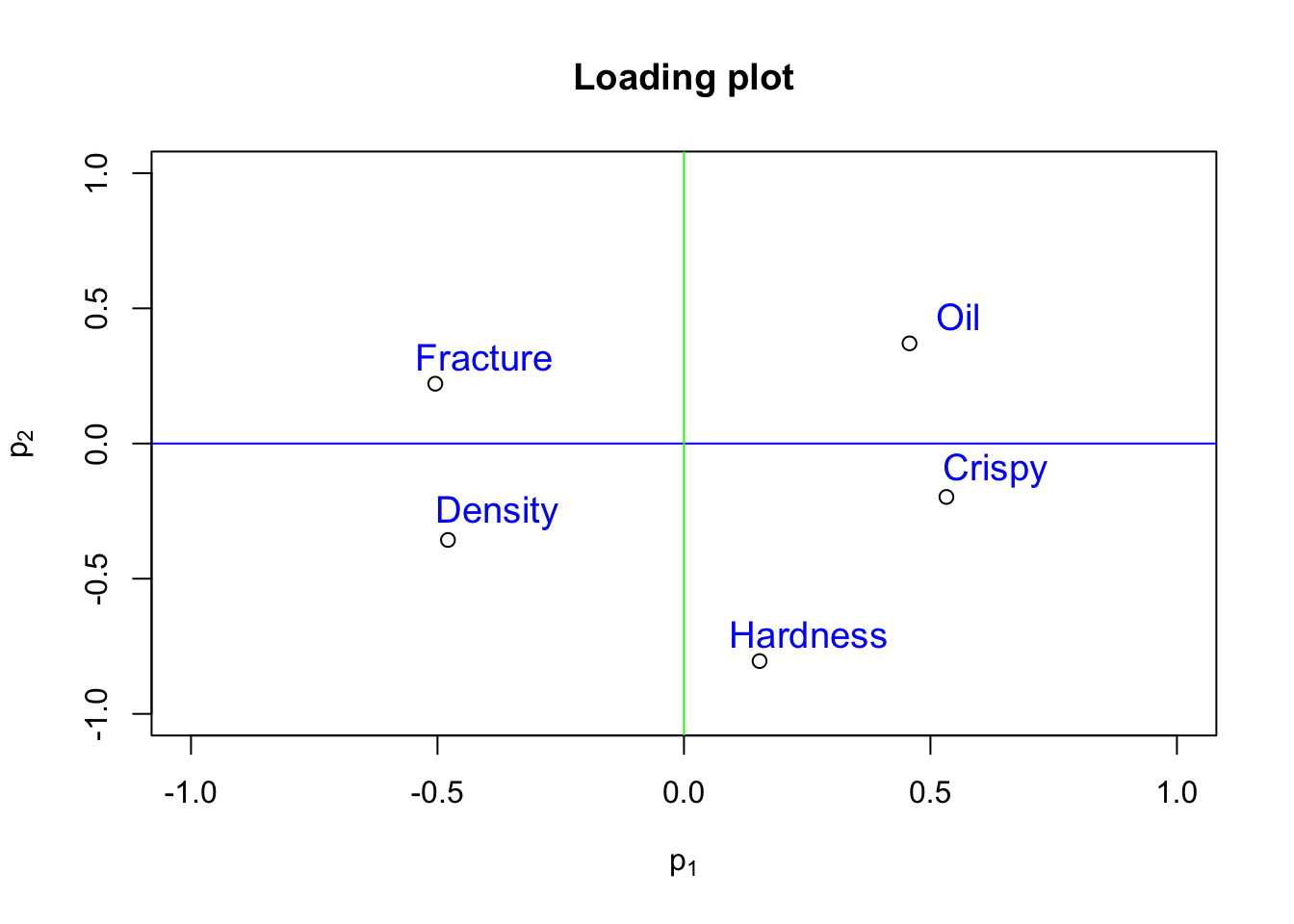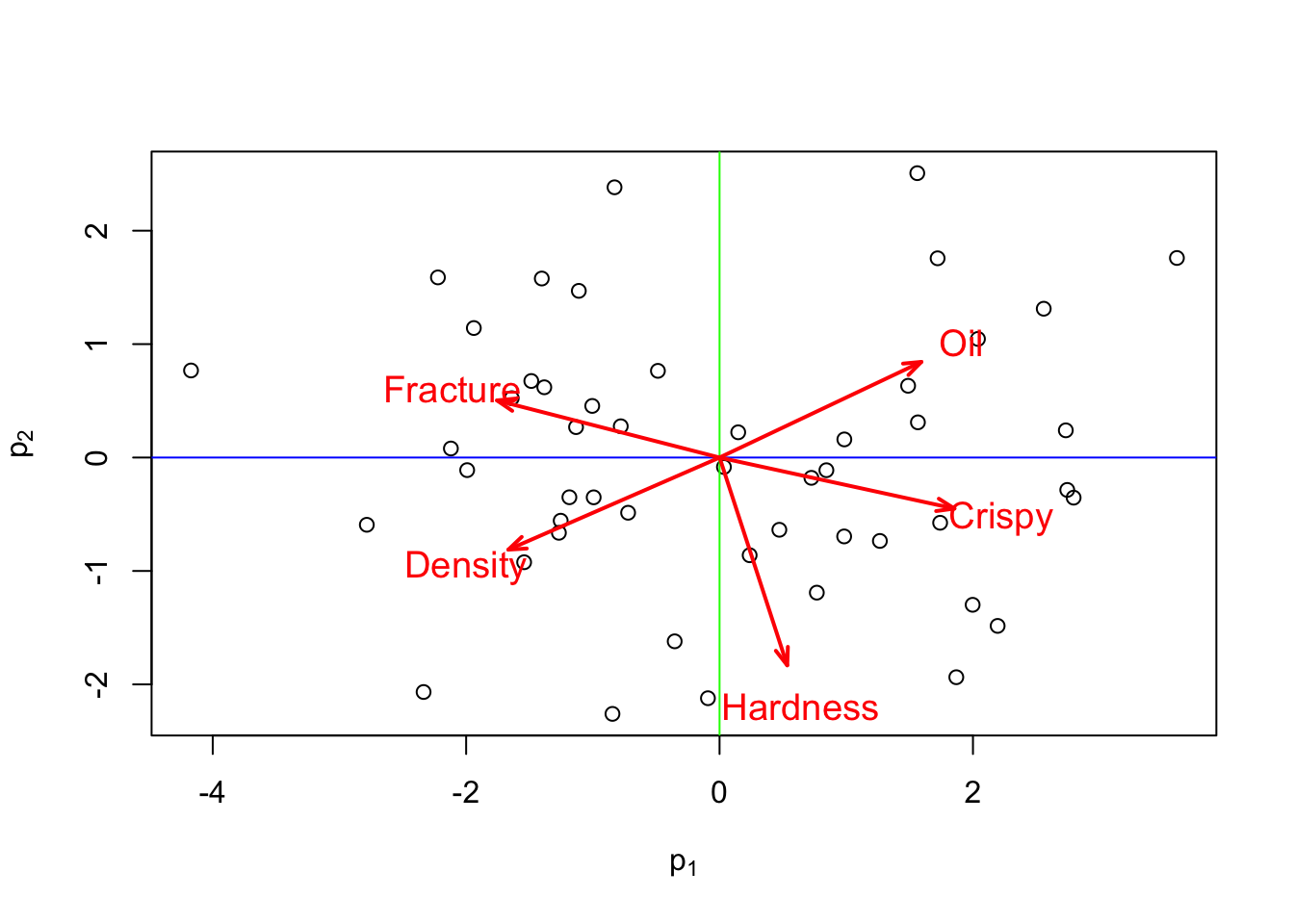#### Interpreting score plots

Let us recall what a score value is: There is one score value for each observation (row) in the data set, so there are $$n$$ score values for the first component, another $$n$$ score values for the second component, and so on. The score value for an observation is the point where that observation projects onto the direction vector for say the first component. Or in vector terminology it is the distance from the origin, along the direction (loading vector) of the first component, up to the point where that observation projects onto the direction vector.

An important point with PCA is that because the matrix $$\mathbf P$$ is orthonormal, any relationships that were present in $$\mathbf X$$ are still present in $$\mathbf Z$$. Thus score plots allow us to rapidly locate similar observations, clusters, outliers and time-based patterns (Dunn 2016).

The first two score vectors, $$\mathbf Z_1$$ and $$\mathbf Z_2$$, explain the greatest variation in the data, hence we usually start by looking at the $$\{\mathbf Z_1, \mathbf Z_2\}$$ scatter plot of the scores.

# load data from previous sections

food.pca.eigen <- eigen(cov(food.pca))
pca.loading <- food.pca.eigen$vectors[,1:2] # select the first two principal components pca.scores <- food.pca %*% pca.loading rownames(pca.scores) <- seq(1, nrow(pca.scores)) # Plot the scores plot(pca.scores, xlab = expression('Z'), ylab = expression('Z'), main = 'Score plot') abline(h = 0, col = "blue") abline(v = 0, col = "green") # Plot the scores as points text(pca.scores[,1]+0.2, pca.scores[,2], rownames(pca.scores), col="blue", cex=0.6)• Points close the average appear at the origin of the score plot. An observation that is at the mean value for all $$k$$-variables will have a score vector $$Z_i = [0, 0,... , 0]$$. • Scores further out are either outliers or naturally extreme observations. • Original observations in $$\mathbf X$$ that are similar to each other will be similar in the score plot, while observations much further apart are dissimilar. It is much easier to detect this similarity in an $$k$$-dimensional space than the original $$d$$-dimensional space, when $$d \gg k$$. #### Interpreting loading plots The loadings plot is a plot of the direction vectors that define the model. They show how the original variables contribute to creating the principal component. loading.vector <- food.pca.eigen$vectors

xlab = expression('p'),
ylab = expression('p'),
ylim = c(-1,1),
xlim = c(-1,1))
abline(h = 0, col = "blue")
abline(v = 0, col = "green")

col="blue", cex=1.2)• Variables which have little contribution to a direction have almost zero weight in that loading.
• Variables which have roughly equal influence on defining a direction are correlated with each other and will have roughly equal numeric weights.
• Strongly correlated variables, will have approximately the same weight value when they are positively correlated. In a loadings plot of e.g. $$p_1$$ vs $$p_2$$ they will appear near each other, while negatively correlated variables will appear diagonally opposite each other.
• Signs of the loading variables are useful to compare within a direction vector; but these vectors can be rotated by 180° and still have the same interpretation.

#### Interpreting Biplots

The biplot is a very popular way for visualization of results from PCA, as it combines both the principal component scores and the loading vectors in a single biplot display.

# Correlation BiPlot
pca.sd <- sqrt(food.pca.eigen$values) # standardize to sd = 1 loading.vector <- food.pca.eigen$vectors

# Plot
plot(pca.scores,
xlab = expression('p'),
ylab = expression('p'))
abline(h = 0, col = "blue")
abline(v = 0, col = "green")

# This is to make the size of the lines more apparent
factor <- 0.5

# Plot the variables as vectors
length = 0.1,
lwd=  2,
angle = 20,
col = "red")

# Plot annotations
col = "red",
cex = 1.2)The plot shows the observations as points in the plane formed by two principal components (synthetic variables). Like for any scatterplot we may look for patterns, clusters, and outliers.

In addition to the observations the plot shows the original variables as vectors (arrows). They begin at the origin $$[0,0]$$ and extend to coordinates given by the loading vector (see loading plot above). These vectors can be interpreted in three ways (Rossiter 2014):

• The orientation (direction) of the vector, with respect to the principal component space, in particular, its angle with the principal component axes: the more parallel to a principal component axis is a vector, the more it contributes only to that PC.

• The length in the space; the longer the vector, the more variability of this variable is represented by the two displayed principal components; short vectors are thus better represented in other dimension.

• The angles between vectors of different variables show their correlation in this space: small angles represent high positive correlation, right angles represent lack of correlation, opposite angles represent high negative correlation.